# Data Structures Programming Finishing up Arrays and Linked

• Slides: 18Data Structures & Programming Finishing up Arrays and Linked Lists Golnar Sheikhshab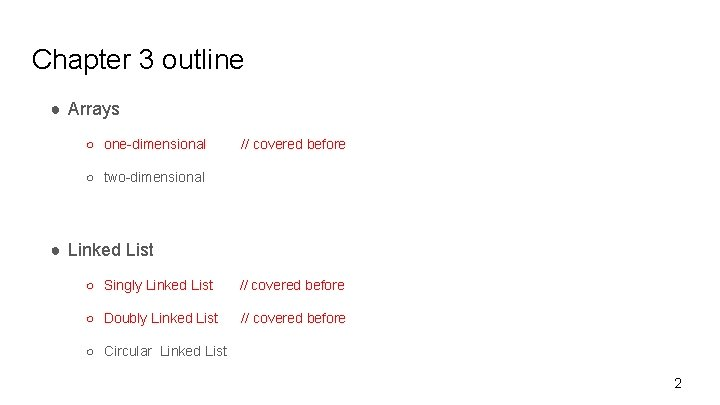Chapter 3 outline ● Arrays ○ one-dimensional // covered before ○ two-dimensional ● Linked List ○ Singly Linked List // covered before ○ Doubly Linked List // covered before ○ Circular Linked List 2Arrays - two dimensional //Array of arrays - also called matrix sometimes const int max_row_num = 10; const int max_col_num = 20; int A[max_row_num][max_col_num]; // dynamic array - allocating memory int** B = new int*(max_row_num); for (int i=0; i< max_row_num; i++ ) B[i] = new int[max_col_num]; // dynamic array -releasing memory for (int i=0; i< max_row_num; i++ ) delete [] B[i]; delete [] B; 3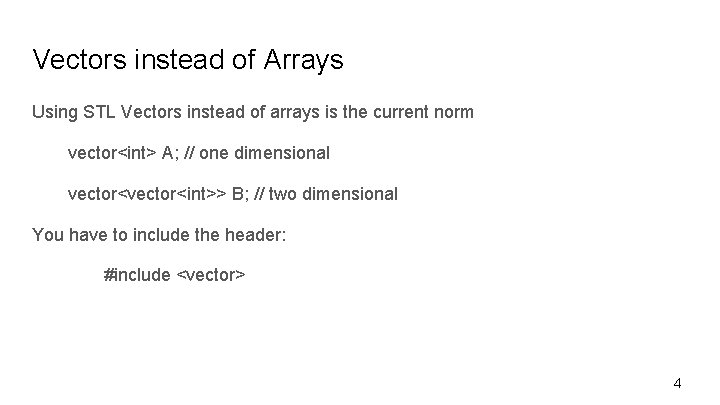Vectors instead of Arrays Using STL Vectors instead of arrays is the current norm vector<int> A; // one dimensional vector<int>> B; // two dimensional You have to include the header: #include <vector> 42 D arrays and positional games 5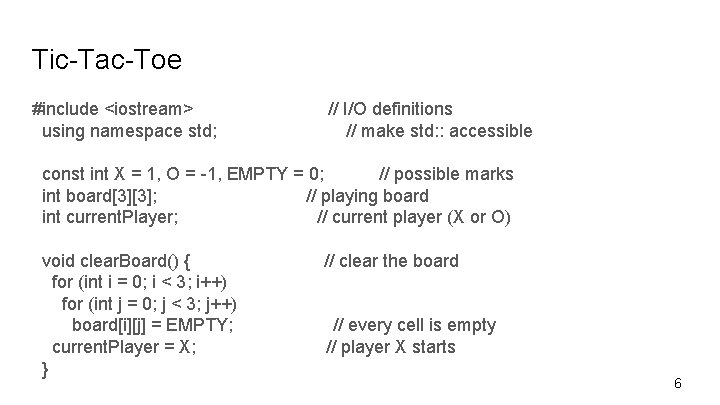Tic-Tac-Toe #include <iostream> using namespace std; // I/O definitions // make std: : accessible const int X = 1, O = -1, EMPTY = 0; // possible marks int board; // playing board int current. Player; // current player (X or O) void clear. Board() { for (int i = 0; i < 3; i++) for (int j = 0; j < 3; j++) board[i][j] = EMPTY; current. Player = X; } // clear the board // every cell is empty // player X starts 6Tic-Tac-Toe (2) void put. Mark(int i, int j) { // mark row i column j board[i][j] = current. Player; // mark with current player current. Player = -current. Player; // switch players } int get. Winner() { if (is. Win(X)) return X; else if (is. Win(O)) return O; else return EMPTY; } // who wins? (EMPTY means tie) 7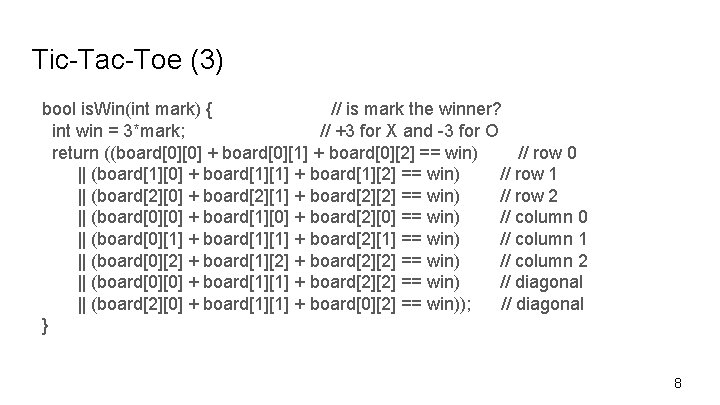Tic-Tac-Toe (3) bool is. Win(int mark) { // is mark the winner? int win = 3*mark; // +3 for X and -3 for O return ((board + board + board == win) // row 0 || (board + board + board == win) // row 1 || (board + board + board == win) // row 2 || (board + board + board == win) // column 0 || (board + board + board == win) // column 1 || (board + board + board == win) // column 2 || (board + board + board == win) // diagonal || (board + board + board == win)); // diagonal } 8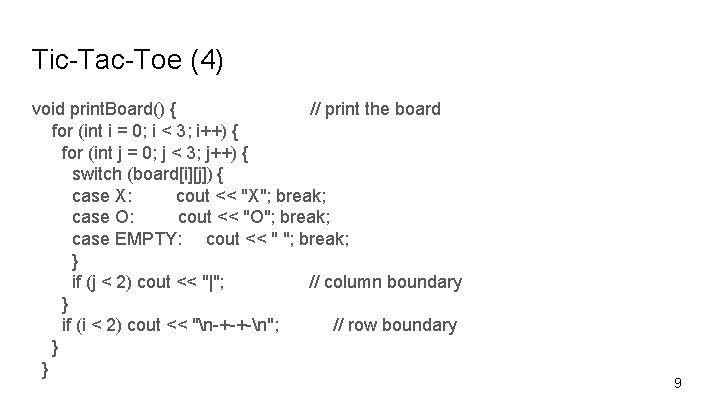Tic-Tac-Toe (4) void print. Board() { // print the board for (int i = 0; i < 3; i++) { for (int j = 0; j < 3; j++) { switch (board[i][j]) { case X: cout << "X"; break; case O: cout << "O"; break; case EMPTY: cout << " "; break; } if (j < 2) cout << "|"; // column boundary } if (i < 2) cout << "n-+-+-n"; // row boundary } } 9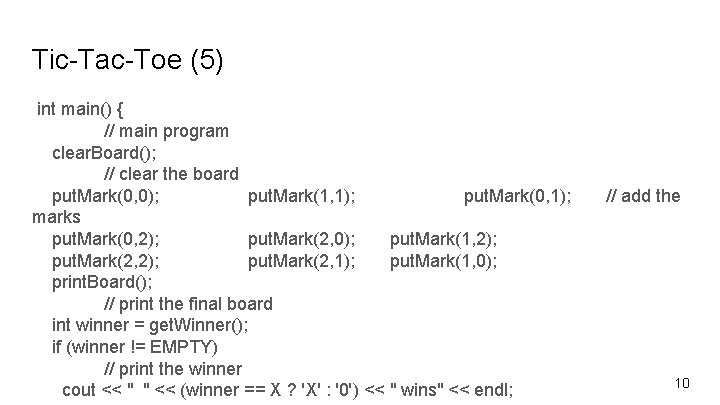Tic-Tac-Toe (5) int main() { // main program clear. Board(); // clear the board put. Mark(0, 0); put. Mark(1, 1); put. Mark(0, 1); marks put. Mark(0, 2); put. Mark(2, 0); put. Mark(1, 2); put. Mark(2, 1); put. Mark(1, 0); print. Board(); // print the final board int winner = get. Winner(); if (winner != EMPTY) // print the winner cout << " " << (winner == X ? 'X' : '0') << " wins" << endl; // add the 10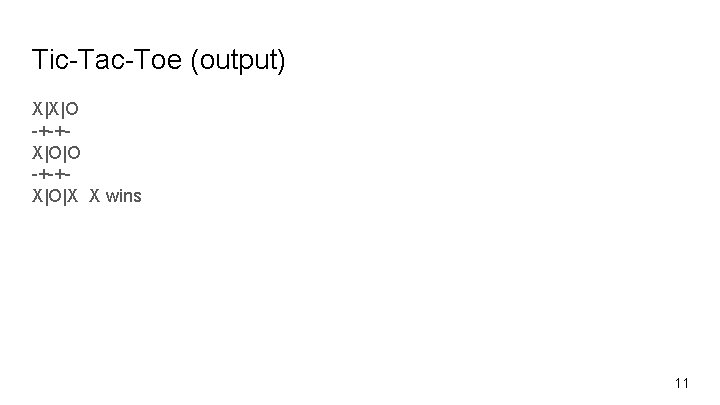Tic-Tac-Toe (output) X|X|O -+-+X|O|X X wins 11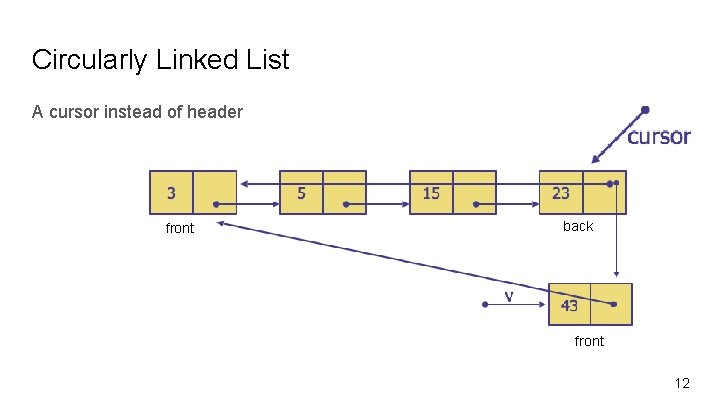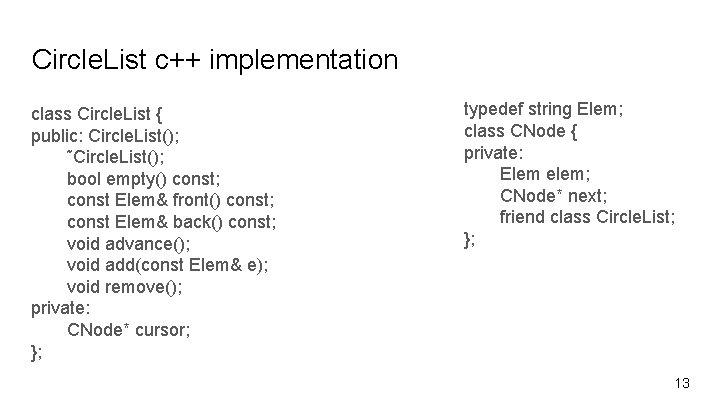Circle. List c++ implementation class Circle. List { public: Circle. List(); ˜Circle. List(); bool empty() const; const Elem& front() const; const Elem& back() const; void advance(); void add(const Elem& e); void remove(); private: CNode* cursor; }; typedef string Elem; class CNode { private: Elem elem; CNode* next; friend class Circle. List; }; 13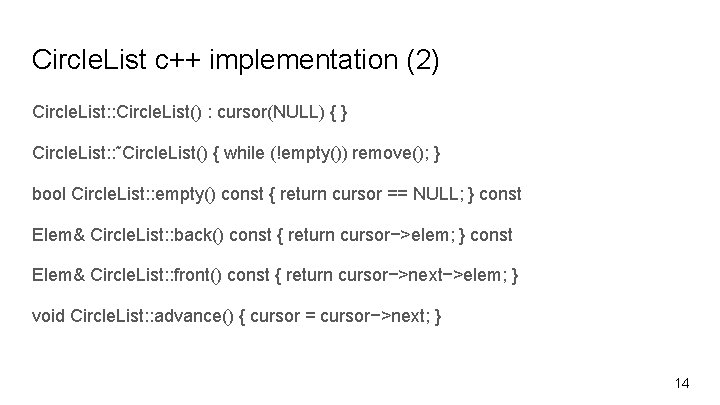Circle. List c++ implementation (2) Circle. List: : Circle. List() : cursor(NULL) { } Circle. List: : ˜Circle. List() { while (!empty()) remove(); } bool Circle. List: : empty() const { return cursor == NULL; } const Elem& Circle. List: : back() const { return cursor−>elem; } const Elem& Circle. List: : front() const { return cursor−>next−>elem; } void Circle. List: : advance() { cursor = cursor−>next; } 14Circle. List c++ implementation (3) void Circle. List: : add(const Elem& e) { CNode* v = new CNode; v−>elem = e; if (cursor == NULL) { v−>next = v; cursor = v; } else { v−>next = cursor−>next; cursor−>next = v; } } 15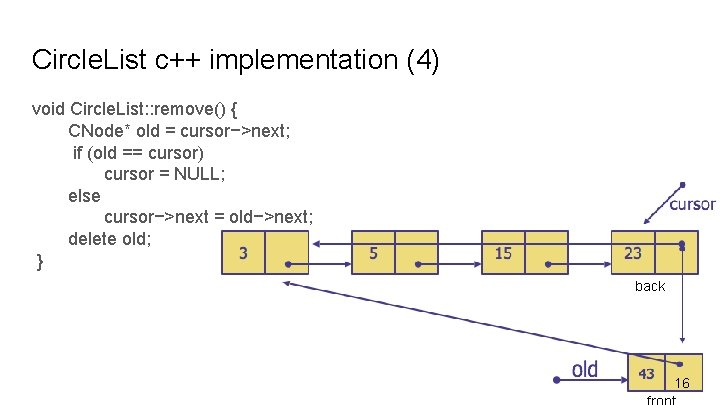Circle. List c++ implementation (4) void Circle. List: : remove() { CNode* old = cursor−>next; if (old == cursor) cursor = NULL; else cursor−>next = old−>next; delete old; } back 16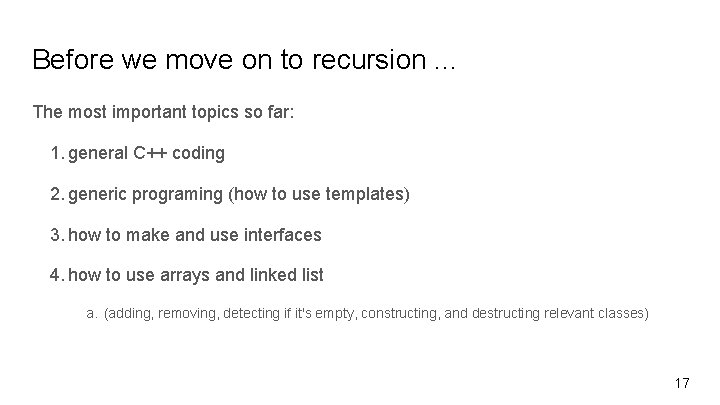Before we move on to recursion. . . The most important topics so far: 1. general C++ coding 2. generic programing (how to use templates) 3. how to make and use interfaces 4. how to use arrays and linked list a. (adding, removing, detecting if it's empty, constructing, and destructing relevant classes) 17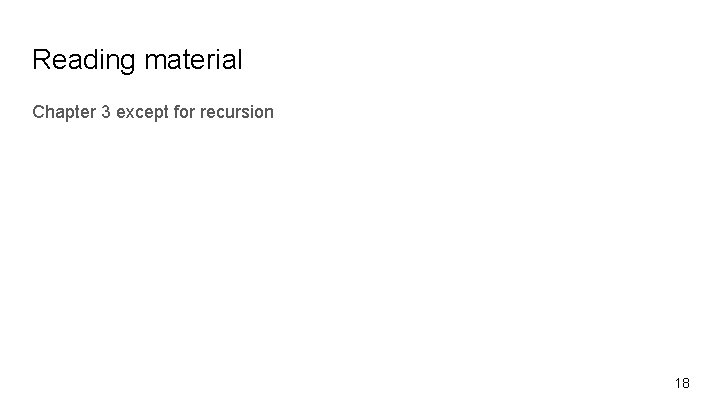Reading material Chapter 3 except for recursion 18